# KSEEB SSLC Class 10 Maths Solutions Chapter 2 Triangles Ex 2.5

KSEEB SSLC Class 10 Maths Solutions Chapter 2 Triangles Ex 2.5 are part of KSEEB SSLC Class 10 Maths Solutions. Here we have given Karnataka SSLC Class 10 Maths Solutions Chapter 2 Triangles Exercise 2.5.

## Karnataka SSLC Class 10 Maths Solutions Chapter 2 Triangles Exercise 2.5

Question 1.
Sides of triangles are given below. Determine which of them are right triangles. In case of a right triangle, write the length of its hypotenuse.
i) 7 cm, 24 cm, 25 cm,
ii) 3 cm, 8 cm, 6 cm.
iii) 50 cm, 80 cm, 100 cm.
iv) 13 cm, 12 cm, 5 cm.
Solution:
In ⊥∆ABC, ∠B = 90°.
Let AB = a, BC = b, Hypotenuse AC = c then
AC2 = AB2 + BC2
c2 = a2 + b2
∴ Here diagonal is the greatest side.
i) a, b,c
7 cm, 24 cm, 25 cm,
c2 = a2 + b2
252 = (7)2 + (24)2
625 = 49 + 576
625 = 625
625 = 49 + 576 625 = 625
∴ This is right angled triangle.
Measurement of Hypotenuse, c = 25 cm.

ii) a c b
3 cm, 8 cm, 6 cm.
c2 = a2 + b2
82 = (3)2 + (6)2
64 = 9 + 36
64 ≠ 45
∴ These are not sides of right angled triangle.

iii) a b c
50 cm, 80 cm, 100 cm.
c2 = a2 + b2
1002= (50)2 + (80)2
10000 = 2500 + 6400
10000 ≠ 8900
∴ These are not sides of right angled triangle.

iv) a b c
12 cm, 5 cm, 13 cm,
c2 = a2 + b2
132 = (12)2 + (5)2
169 = 144 + 25
169 = 169
∴ These are sides of right angled triangle.
Measurement of Hypotenuse =13 cm.

Question 2.
PQR is a triangle right angled at P and M is a point on QR such that PM ⊥ QR. Show that PM2 = QM.MR.
Solution:Data: PQR is a triangle 9 right angled at P and M is a point on QR such that PM ⊥ QR.
To Prove: PM2 = QM.MR
Proof: In ⊥D QPR, ∠P = 90°,
QR2 = QP2 + PR2 ……………. (i)
In ⊥D PMQ, ∠M = 90°
QP2 = PM2 + QM2 ………. (ii)
In ⊥D PMR, ∠M = 90°
PR2 = PM2 + MR2 ……………. (iii)
By Adding eqn. (ii) and (iii)
QP2 + PR2 = PM2 + QM2 + PM2 + MR2
QR2 = 2PM2 + QM2 + MR2 (. Eqn. (i))
(QM + MR)2 = 2PM2 + QM2 + MR2
QM2 + MR2 + 2QM.MR = 2PM2 + QM2 + MR2
QM2 – QM2 + MR2 – MR2 + 2QM.MR = 2PM2
2QM.MR = 2PM2
2PM2 = 2QM.MR
∴ PM2 = QM.MR

Question 3.
In the following figure, ABD is a triangle right angled at A and AC ⊥BD. Show that
i) AB2= BC.BD
ii) AC2 = BC.DC
iii) AD2 = BD.CDSolution:
Data: In ∆ABD, ∠A = 90°,
AC ⊥ BD.
To Proved: AB2 = BC.BD
ii) AC2 = BC.DC
iii) AD2 = BD.CD

i) AB2 = BC.BD
∆ACB ~ ∆BAD (. Theorem7)
$$\frac{\mathrm{AB}}{\mathrm{BD}}=\frac{\mathrm{BC}}{\mathrm{AB}}$$
∴ AB2= BC × BD.

ii) AC2 = BC.DC
∆BCA ~ ∆ACD
$$\frac{\mathrm{AB}}{\mathrm{AD}}=\frac{\mathrm{AC}}{\mathrm{CD}}=\frac{\mathrm{BC}}{\mathrm{AC}}$$
∴ AC × AC = BC × CD
∴ AC2 = BC × CD

iii) AD2 = BD.CD
$$\frac{\mathrm{AD}}{\mathrm{BD}}=\frac{\mathrm{CD}}{\mathrm{AD}}=\frac{\mathrm{AC}}{\mathrm{AB}}$$
∴ AD × AD = BD × DC
∴ AD2 = BD × DC

Question 4.
ABC is an isosceles triangle right angled at C. Prove that AB2 = 2AC2.
Solution:
Data: ABC is an isosceles triangle right angled at C.To Prove: AB2 = 2AC2.
In ⊥∆ACB, ∠C = 90°
∴ AB2= AC2 + BC2
(∵ Pythagoras Theorem)
AB2 = AC2 + AC2 (∵ AC = BC)
AB2 = 2 AC2.

Question 5.
ABC is an isosceles triangle with AC = BC. If AB2 = 2AC2, prove that ABC is a right-angled triangle.
Solution:
Data: ABC is an isosceles triangle with AC = BC.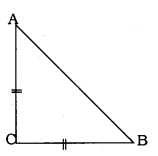AB2 = 2 AC2.
To Prove: ∆ABC is a right angled triangle
AB2 = 2AC2 (Data)
AB2 = AC2 + AC2
AB2 = AC2 + BC2 (∵ AC = BC)
Now, in ∆ABC, square of one side is equal to squares of other two sides.
∆ABC is a right angled triangle, Opposite angle to AB, i.e., ∠C is 90°.

Question 6.
ABC is an equilateral triangle of side 2a. Find each of its altitudes.
Solution:
Data: ABC is an equilateral triangle of side 2a.To Prove: Altitude of ∆ABC,
In equilateral triangle
D bisect base.
∴ AB = BC = CA = 2a. 3 D a
If BC = 2a,
$$\frac{1}{2} \mathrm{BC}=\mathrm{a}$$ unit
∴ BD = DC = a.
Now, in ⊥∆ADB, ∠D = 90°
∴ AD2 + BD2 = AB2
AD2 + a2 = (2a)2
AD2 + a2 = 4a2
∴ AD2 = 4a2 – a2
$$\sqrt{A D^{2}}=\sqrt{3 a^{2}}$$
∴Altitude,$$\mathrm{AD}=\sqrt{3} \mathrm{a}$$ unit.

Question 7.
Prove that the sum of the squares of the sides of a rhombus is equal to the sum of the squares of its diagonals.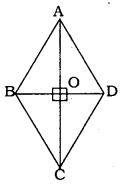Solution:
Data: ABCD is a rhombus.
Here, AB = BC = CD = DA.
Diagonals AC and BD intersects at ’O’.
To Prove: AB2 + BC2 + CD2 + DA2 = AC2 + BD2.
In rhombus diagonals bisects perpendicularly.
∴ ∠AOB = ∠AOD = 90°.
In ⊥∆AOB,
AB2 = AO2 + BO2 ……..(i)
In ⊥∆BOC,
AC2 = BO2 + CO2 ……..(ii)
In ⊥∆COD,
CD2 = OC2 + OD2 ……….(iii)
In ⊥∆AOD,
AD2 = AO2 + OD2…………(iv)
By Adding equations (i) + (ii) + (iii) + (iv)
AB2 + BC2 + CD2 + DA2 =
= AO2 + BO2 + BO2 + CO2 + CO2 + DO2 + AO2 + OD2
= 2AO2 + 2BO2 + 2CO2 + 2DO2
= 2AO2 + 2CO2 + 2BO2 + 2DO2
Now, RHS = 2AO2 + 2CO2 + 2BO2 + 2DO2
$$=2 \times\left(\frac{1}{2} \mathrm{AC}\right)^{2}+2 \times\left(\frac{1}{2} \mathrm{AC}\right)^{2}+2 \times\left(\frac{1}{2} \mathrm{BD}\right)^{2}+2 \times\left(\frac{1}{2} \mathrm{BD}\right)^{2}$$
$$=2 \times \frac{1}{4} \mathrm{AC}^{2}+2 \times \frac{1}{4} \mathrm{AC}^{2}+2 \times \frac{1}{4} \mathrm{BD}^{2}+2 \times \frac{1}{4} \mathrm{BD}^{2}$$
$$=\frac{1}{2} A C^{2}+\frac{1}{2} A C^{2}+\frac{1}{2} B D^{2}+\frac{1}{2} B D^{2}$$
RHS = AC2 + BD2
∴ LHS = RHS
∴ AB2 + BC2 + CD2 + DA2 = AC2 + BD2.

Question 8.
In the following figure, O is a point in the interior of a triangle ABC, OD ⊥ BC, OE ⊥ AC, OF ⊥ AB. Show that
i) OA2 + OB2 + OC2 – OD2 – OE2 – OF2 = AF2 + BD2 + CE2
ii) AF2 + BD2 + CE2 = AE2 + CD2 + BF2.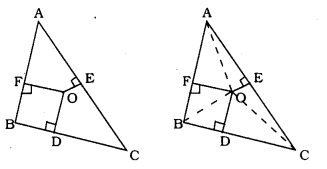Solution:
Data: O is a point in the interior of a triangle ABC, OD ⊥ BC, OE ⊥ AC, OF⊥ AB.
To Proved: i) OA2 + OB2 + OC2 – OD2 – OE2 – OF2 = AF2 + BD2 + CE2
ii) AF2 + BD2 + CE2 = AE2 + CD2 + BF2

(i) OA2 + OB2 + OC2 – OD2 – OE2 – OF2 = AF2 + BD2 + CE2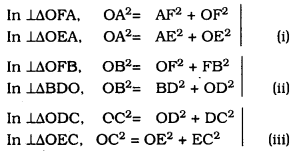OA2 = AF2 + OF2 → (i)
OB2 = BD2 + OD2 → (ii)
OC2 = OE2 + EC2 → (iii)
By Adding equations (i) + (ii) + (iii),
OA2 + OB2+ OC2 = AF2 + OF2 + BD2 + OD2 + OE2 +EC2
∴ OA2 + OB2 + OC2 – OE2 – OF2 – OD2 = AF2 + BD2 + CE2

(ii) AF2 + BD2 + CE2 = AE2 + CD2 + BF2.
OA2 = AF2 + OF2
∴ AF2 = OA2 – OF2 → (i)
OB2 = BD2 + OD2
∴BD2 = OB2 – OD2 → (ii)
OC2 = OE2 + EC2
∴ CE2 = OC2 – OE2 → (iii)
From adding equations (i) + (ii) + (iii),
AF2 + BD2 + CE2 = OA2 – OF2 + OB2 – OD2 + OC2 – OE2
AF2 + BD2 + CE2 = OA2 – OE2 + OB2 – OF2 + OC2 – OE2
∴ AF2 + BD2 + CE2 = AE2 + FB2 + CD2 .

Question 9.
A ladder 10 m long reaches a window 8 m above the ground. Find the distance of the foot of the ladder from base of the wall.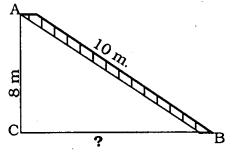Solution:
In ⊥∆ACB, ∠C = 90°, BC = ?
AC2 + CB2 = AB2
(8)2 + CB2 = (10)2
64 + CB2 = 100
CB2 = 100 – 64
CB2 = 36
∴ CB = 6
∴ Ladder is at a distance of 6m from the base of the wall.

Question 10.
A guy wire attached to a vertical pole of height 18 m is 24 m long and has a stake attached to the other end. How far from the base of the pole should the stake be driven so that the wire will be taut?Solution:
In ⊥∆PQR, ∠Q = 90°, QR = ?
∴ PQ2 + QR2 = PR2
(18)2 + QR2 = (24)2
324 + QR2 = 576
QR2 = 576 – 324
QR2 = 252
∴ QR = 15.8 m.

Question 11.
An aeroplane leaves an airport and flies due north at a speed of 1000 km per hour. At the same time, another aeroplane leaves the same airport and flies due west at a speed of 1200 km per hour. How far apart will be the two planes after $$1 \frac{1}{2}$$ hours?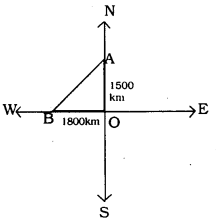Solution:
Distance travelled by aeroplace trowards North is $$1 \frac{1}{2}$$ hours :
$$=1000 \times 1 \frac{1}{2}$$
$$=1000 \times \frac{3}{2}$$
= 1500 km.
Diatance travelled by aeroplane towards West in $$1 \frac{1}{2}$$ Hours :
$$=1200 \times 1 \frac{1}{2}$$
$$=1200 \times \frac{3}{2}$$
= 1800 km.
In ⊥∆AOB,
AB2 = OA2 + OB2
= (1500)2 + (1800)2
= 2250000 + 3240000 = 5490000
$$\mathrm{AB}=\sqrt{5490000}$$
$$A B=\sqrt{90000 \times 61}$$
$$A B=300 \sqrt{61} \mathrm{km}$$ km
∴ Two planes are 300V6T km. apart after 14 hours

Question 12.
Two poles of heights 6 m and 11m stand on a plane ground. If the distance between the feet of the poles is 12 m, find the distance between their tops.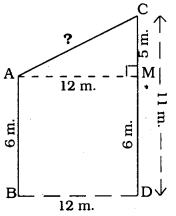Solution:
Pole AB = 6 m.
Pole CD = 11 m.
Distance between poles BD = 12 m.
Distance between feet of the poles, AC = ?
ABDM is a rectangle, AB = MD = 6 m.
BD = AM = 12 m.
In ⊥∆AMC, ∠AMC = 90°
AM = 12 m, CM = 5 m. AC = ?
AC2 = AM2 + CM2
= (12)2 + (5)2
= 144 + 25
AC2 = 169
∴ AC = 13 m.

Question 13.
D and E are points on the sides CA and CB respectively of a triangle ABC right angled at C.
Prove that AE2 + BD2 = AB2 + DE2.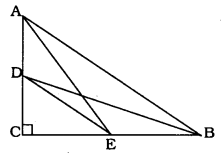Solution:
Data: In ∆ABC, ∠C = 90°, D and E are points on the sides CA and CB respectively
Top Prove: AE2 + BD2 = AB2 + DE2
In ⊥∆ACE, ∠C = 90°
∴ AE2 = AC2 + CE2 ………. (i)
In ⊥∆DEB, ∠C = 90°
∴ BD2 = DC2 + CB2 ………… (ii)
From adding equations (i) + (ii)
AE2 + BD2 = AC2 + CE2 + DC2 + CB2
= AC2 + CB2 + DC2 + CE2
∴ AE2 + BD2 = AB2 + DE2 ( . Theorem 8).

Question 14.
The perpendicular from A on side BC of a ∆ABC intersects BC at D such that DB = 3CD (see the following figure) Prove that 2AB2 = 2AC2 + BC2.Solution:
In ∆ACD,
AC2 = AD2 + DC2
AD2 = AC2 – DC2……….. (1)
In ∆ABD,
AB2 = AD2 + DB2
AD2 = AB2 – DB2………. (2)
From equations (1) and (2),
AC2 – DC2 = AB2 – DB2 ………… (3)
3DC = DB (data given)
$$\mathrm{DC}=\frac{\mathrm{BC}}{4}, \text { and } \mathrm{DB}=\frac{3 \mathrm{BC}}{4}$$ ……….. (3)
Substituting eqn. (4) in eqn. (3),
$$\mathrm{AC}^{2}-\left(\frac{\mathrm{BC}}{4}\right)^{2}=\mathrm{AB}^{2}-\left(\frac{3 \mathrm{BC}}{4}\right)^{2}$$
$$A C^{2}-\frac{B C^{2}}{16}=A B^{2}-\frac{9 B C^{2}}{16}$$
$$\frac{16 \mathrm{AC}^{2}-\mathrm{BC}^{2}}{16}=\frac{16 \mathrm{AB}^{2}-9 \mathrm{BC}^{2}}{16}$$
16AC2 – BC2 = 16 AB2 – 9BC2
16AB2 – 16AC2 = 9 BC2 – BC2
16AB2 – 16AC2 = 8BC2
8(2AB2 – 2AC2 = BC2)
2AB2 – 2AC2 = BC2
2AB2 = 2AC2 ≠ BC2

Question 15.
In an equilateral triangle ABC, D is a point on side BC such that $$B D=\frac{1}{3} B C$$, Prove that 9AD2 = 7AB2.
Solution:
∆ABC is an equilateral triangle.AB = BC = AC = a
In ∆ABC, AE is perpendicular line.
$$B E=E C=\frac{B C}{2}$$
Altitude, $$\mathrm{AE}=\frac{\mathrm{a} \sqrt{3}}{2}$$
$$\mathrm{BD}=\frac{1}{3} \mathrm{BC}(\mathrm{Data})$$
$$B D=\frac{a}{3}$$
DE = BE – AD
$$=\quad \frac{a}{2}-\frac{a}{3}=\frac{a}{6}$$
AD2 = AE2 + DE2
$$=\left(\frac{\mathrm{a} \sqrt{3}}{2}\right)^{2}+\left(\frac{\mathrm{a}}{6}\right)^{6}$$
$$=\frac{3 a^{2}}{4}+\frac{a^{2}}{36}=\frac{27 a^{2}+a^{2}}{36}$$
$$=\frac{28 \mathrm{a}^{2}}{36}$$
$$=\frac{7}{9} \mathrm{a}^{2}$$
$$\mathrm{AD}^{2}=\frac{7}{9} \mathrm{AB}^{2} \quad 9 \mathrm{AD}^{2}=7 \mathrm{AB}^{2}$$

Question 16.
In an equilateral triangle, prove that three times the square of one side is equal to four times the square of one of its altitudes.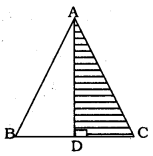Solution:
Data: ABC is an equilateral triangle.
Here, AB = BC = CD.
To Prove: 3AC2 = 4AD2
∴ AC2 = AD2 + DC2
$$=A D^{2}+\left(\frac{1}{2} A C\right)^{2}$$
$$\left( \begin{array}{l}{\mathrm{DC}=\frac{1}{2} \mathrm{BC}} \\ {\mathrm{DC}=\frac{1}{2} \mathrm{AC}}\end{array}\right)$$
$$\mathrm{AC}^{2}=\mathrm{AD}^{2}+\frac{1}{4} \mathrm{AC}^{2}$$
$$\frac{\mathrm{AC}^{2}}{1}-\frac{1}{4} \mathrm{AC}^{2}=\mathrm{AD}^{2}$$
$$\frac{4 \mathrm{AC}^{2}-1 \mathrm{AC}^{2}}{4}=\mathrm{AD}^{2}$$
∴ 3AC2 = 4AD2.

Question 17.
Tick the correct answer and justify:
In ∆ABC, $$A B=6 \sqrt{3} \mathrm{cm}$$. AC = 12 cm. and BC = 6 cm. The angle B is
A) 120°
b) 60°
C) 90°
D) 45°
Solution:
C) 90°Justification:
a             b           c
$$6 \sqrt{3}$$        6             12
Here largest side is 12 cm.
If square of the hypotenuse is equal to square of other two sides, then it is a right angled triangle.
∴ c2 = a2 + b2
AC2 = AB2 + BC2
$$(12)^{2}=(6 \sqrt{3})^{2}+(6)^{2}$$
144 = 36 × 3 + 36
144 = 108 + 36
144 = 144
∴ ∆ABC is a right angled triangle and angle opposite to hypotenuse, ∠B = 90°.

We hope the given KSEEB SSLC Class 10 Maths Solutions Chapter 2 Triangles Ex 2.5 will help you. If you have any query regarding Karnataka SSLC Class 10 Maths Solutions Chapter 2 Triangles Exercise 2.5, drop a comment below and we will get back to you at the earliest.

error: Content is protected !!